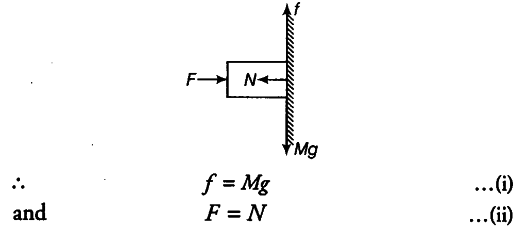# A block of mass M is held against a rough vertical wall by pressing it with a finger

A block of mass M is held against a rough vertical wall by pressing it with a finger. If the coefficient of friction between the block and the wall is p and the acceleration due to gravity is g, calculate the minimum force required to be applied by finger to hold the block against the wall?

Given, mass of the block = M
Coefficient of friction between the block
and the wall = \mu
Let a force F be applied on the block to hold the block against the wall. The normal reaction of mass be N and force of friction acting upward be f.
In equilibrium, vertical and horizontal forces should be balanced separately.But force of friction (f) =\mu N
= $\mu$F [using Eq. (ii)] …(iii)
. m
From Eqs. (i) and (iii), we get
$\mu$F = Mg

or F = Mg/$\mu$F